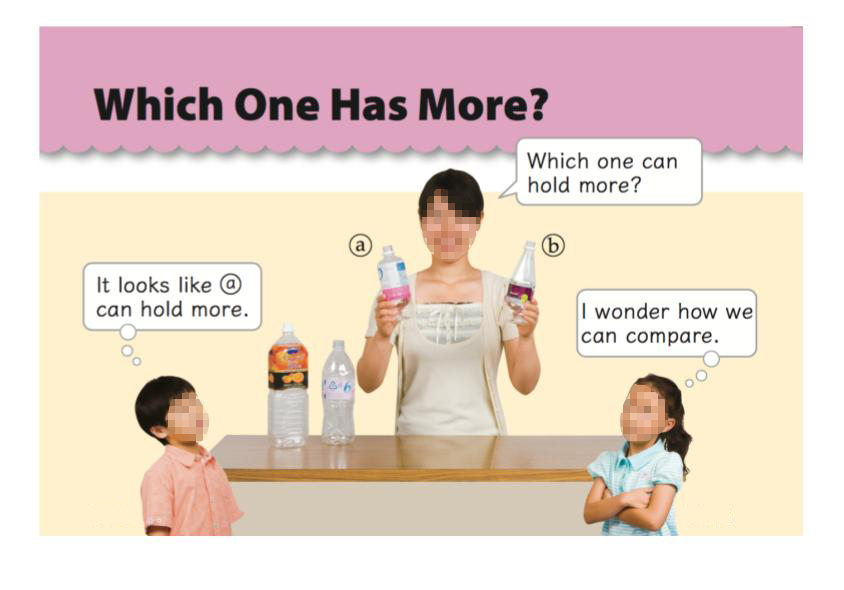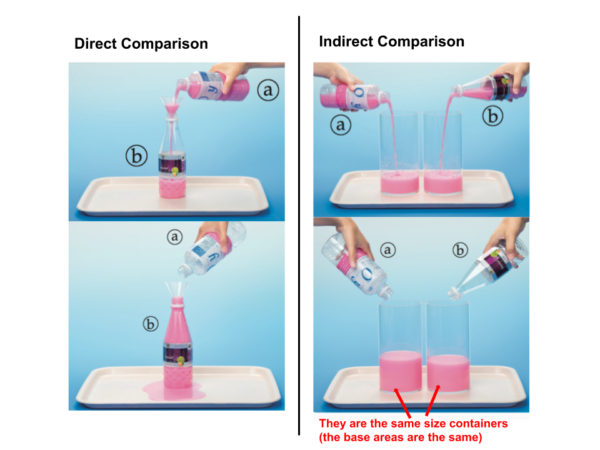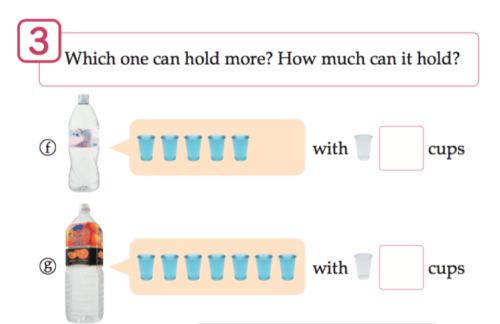# Measuring Liquid Volume

## Overview

In this resource, we discuss the teaching and learning of measuring liquid volume. Specifically, we attend to how students’ understanding of liquid volume might progress through the four stages of measurement. This resource builds on our measurement overview, which we recommend you read first if you have not done so yet.

## Introducing Liquid Volume and Capacity

According to the CCSS, students are expected to learn to measure and estimate liquid volume in Grade 3. So, what is liquid volume and how does it relate to the four stages of measurement?

If volume is the amount of 3-D space contained by a closed surface (e.g. the amount of space within a given cube, or cylinder, or any other 3-D shape), liquid volume is a way to measure an amount of liquid by describing it how much 3-D space it occupies.

Liquid volume is often discussed in the context of a related idea: capacity. Capacity is the maximum amount of liquid a container can hold. Often times, the initial introduction of liquid volume is by comparing the capacities of different containers, as seen in the following excerpt from Mathematics International:Reproduced with permission from Tokyo Shoseki publishing company, Japan. Mathematics International Grade 1, p. 91

## The Four Stages of Measurement with Liquid Volume

Just as with all measurement attributes (e.g. length, weight, etc), students benefit from progressing through the four stages of measurement with liquid volume. Below, we discuss experiences that can help students move through these stages.

### Stage 1: Direct Comparison of Liquid Volumes

One way to directly compare the capacity of two containers is to fill up one container and transfer that amount of water into the other. If it overflows, the first container is bigger, or is able to hold more water. If all of the water from the first container can be poured into the second container without completely filling it, then the second container holds more water.

### Stage 2: Indirect Comparison of Liquid Volumes

One way to indirectly compare the capacities of two containers is to fill up both containers and pour their content into two identical containers. By looking at the height of the liquid in the two containers, we can decide which container had more liquid.Reproduced with permission from Tokyo Shoseki publishing company, Japan. Mathematics International Grade 1, p. 91-92

Importantly, the size of the two containers that receive the content of the original two containers must be identical to make a judgement. We know that many young children judge the amount of liquid in a container based on its level (height of liquid) without paying attention to other dimensions of the container. This is something that teachers need to keep in mind.. Teachers may directly address this misconception by, for example, having students pour out the same amount of liquid into two different containers. Teachers can also address this misconception during the direct comparison stage by helping students realize that a taller container does not always hold more liquid than a shorter one.

This indirect comparison approach then leads to the third stage of measurement: measuring with a non-standard unit.

### Stage 3: Measuring Liquid Volume with Non-Standard Units

What can we do if we cannot find two identical containers that are large enough to hold the entire content of the original two containers? For example, what if we only have one small cup? To help us measure our original containers, we can use the cup as a non-standard unit. We can figure out how many little cups the liquid from each container can fill up and compare the two amounts.Reproduced with permission from Tokyo Shoseki publishing company, Japan. Mathematics International Grade 1, p. 93

There are a couple of important ideas students can develop while measuring with non-standard units. These ideas are not specific to measuring liquid volume, and students have, ideally, discussed these ideas already discussed when learning to measure length.

The first important idea is the inverse relationship between the size of a unit and the number of units. If you measure a given quantity, measuring with a smaller unit will yield a larger number of units than measuring with a larger unit. Making this understanding explicit will help students later on when they must convert measurements between different units. If you are converting a measurement into a larger unit, the number should be less, and vice versa. Another idea students need to understand is that measurements must have the same unit in order to compare them. This idea of having a “common” unit eventually leads students to understand why having standard units is important; it allows people in different locations to communicate measurements.  By seeing that ideas used in measuring length are useful in measuring liquid volume, students begin to understand that these ideas can be generalized to all types of measurement.

### Stage 4: Measuring Liquid Volume with Standard Units

In the CCSS, the unit of liquid volume introduced in Grade 3 is liter (L), which is a standard unit in the metric (or SI) system. Another commonly used unit of liquid volume is milliliter (mL), where 1000 mL = 1 L. Many soda bottles are 500 mL, 1L or 2L. As a result, even though the Metric system has not been widely accepted in the United States, liter and milliliter may be two units that many people are comfortable  with. Interestingly, the CCSS does not specify when milliliter should be introduced. However, a Grade 4 standard (4.MD.2) expects students to be able to solve problems involving milliliters, allowing us to assume that students must be introduced to it in either Grade 3 or 4.

Many Japanese textbooks introduce deciliter (dL) as a unit of liquid volume. This is because liquid volume is only the second measurable attribute studied in the curriculum, and students need to experience measurement as an iteration of a unit. Liter is a relatively large unit, and it is difficult to arrange meaningful experiences where children will actually iterate with liter as a unit. Because it is not practical to introduce the unit of deciliter in the US classrooms, teachers may want to incorporate more activities where students are actually measuring with non-standard (and smaller than 1L) units in Stage 3.

## Discussion Questions

After reading this resource, it may be helpful to discuss the following questions as a team.

1. What do you see as the key understandings students need to develop about measurement of liquid volume?
2. How does your own curriculum develop these ideas?
3. What is difficult for students in moving from direct to indirect comparison and non-standard to standard units?
4. How will your unit design motivate and support students to move from direct comparison to indirect comparison and non-standard to standard units?
5. How will your students use their current understanding of measurement to make sense of liquid measurement?